# AP Board 6th Class Maths Solutions Chapter 2 Whole Numbers InText Questions

AP State Syllabus AP Board 6th Class Maths Solutions Chapter 2 Whole Numbers InText Questions and Answers.

## AP State Syllabus 6th Class Maths Solutions 2nd Lesson Whole Numbers InText QuestionsQuestion 1.
Fill the following table with the successor and predecessor of the numbers provided. (Page No. 15)

 S.No. Natural number Predecessor Successor 1. 135 2. 237 3. 999

Solution:

 S.No. Natural number Predecessor Successor 1. 135 134 136 2. 237 236 238 3. 999 998 1000

Discuss

Question 1.
Which number has no successor ? (Page No. 15)
Each and every number has a successor.

Question 2.
Which number has no predecessor? (Page No. 15)
Zero (0) has no predecessor in the set of whole numbers.

Check Your Progress (Page No. 16)

Question 1.
Which is the smallest whole number?
Zero(0) is the smallest whole number.Let’s Think (Page No. 16)

Question 1.
Are all natural numbers whole numbers? .
Solution:
Yes. All the natural numbers are whole numbers.

Question 2.
Are all whole numbers natural numbers?
Solution:
No. All the whole numbers are not natural numbers.

Let’s Do (Page No. 17)

Show these on number line:

i) 5 + 3
Solution: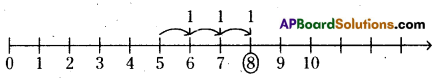Draw the number line starting with ‘O’.
Start from 5, make 3 jumps to the right of 5 on the number line. We reach 8.
So, 5 + 3 = 8

ii) 5 – 3
Solution: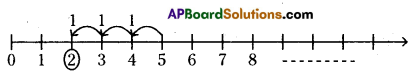Draw the number line starting with zero (0).
Start from 5, make 3 jumps to the left of 5 on the number line, we reach 2.
So, 5 – 3 = 2iii) 3 + 5
Solution: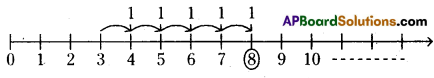Draw the number line starting with zero (0).
Start from 3, we make 5 jumps to the right of 3 on the number line. We reach 8. So, 3 + 5 = 8

iv) 10 + 1
Solution: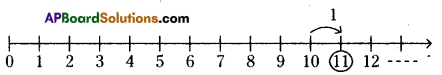Draw the number line which starts with zero (0).
Start from 10, make 1 jump to the right of 10 on the number line. We reach’ll. So, 10 + 1 = 11

v) 8 – 5
Solution: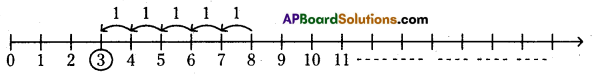Draw the number line which starts with zero (0).
Start from 8, we make 5 jumps to the left of 8 on the number line. We reach 3. So, 8-5 = 3

Let’s Explore (Page No. 17)

Find the following by using number line:

Question 1.
Which number should be deducted from 8 to get 5?
Solution:Draw the number line, which starts with zero (0).
To get 5 from 8. We have to start from 8. We make 3 jumps to the left of 8 on the number line, we reach 5. As we are moving on left side we have minus sign.
So, 8 – 3 = 5
Therefore to get 5 we deduct 3 from 8.Question 2.
Which number should be deducted from 6 to get 1?
Solution: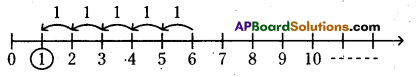Draw the number line starting with zero (0).
To get 1 from 6 we have to start from 6.
We make 5 jumps to the left of 6 on the number line. We reach 1. As we have moved to left side, we have 6-5 = 1 Threfore to get 1 we deduct 5 from 6.

Question 3.
Which number should be added to 6 to get 8?
Solution: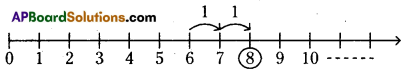Draw the number line starts with zero (0).
To get 8 from 6, we have to start from 6.
We make 2 jumps to the right of 6 on the number line to reach 8; So, 6 + 2 = 8 ‘
Therefore to get 8 we add 2 to 6.

Question 4.
How many 6 are needed to get 30?
Solution: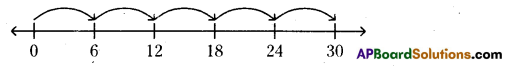Draw the number line starts with zero (0).
Start from 0 and make 6 jumps to the right of the zero as the number line. Now, treat 6 jumps as one step. So, to reach 30, we make 5 steps.
So, 5 × 6 = 30

Question
Raju and Gayatri together made a number line and played a game on it.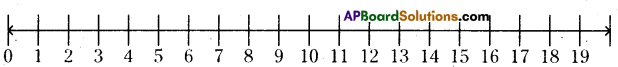Raju asked “Gayatri, where will you reach if you jump thrice, taking leaps of 3, 8 and 5”? Gayatri said the first leap will take me to 3 and then from there I will reach 11 in the second step and another five steps from there to 16′.
Draw Gayatri’s steps and verify her answers.
Play this game using addition and subtraction on this number line with your friend.
Solution: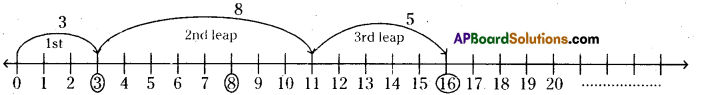Lets Think (Page No. 19)

Question 1.
Are the whole numbers closed under subtraction?
Solution:
8 – 5 = 3, a whole number
5 – 8 = -3 is not a whole number
Therefore whole numbers are not closed under subtraction.

Question 2.
Are the whole numbers closed under division?
Solution:
6 ÷ 3 = 2, a whole number
3 ÷ 6 = $$\frac{3}{6}$$, not a whole number 6
Therefore, whole numbers are not closed under division.

Check Your Progress (Page No. 19)

Question 1.
Find out 12 ÷ 3 and 4 ÷ 7.
Solution:
12 ÷ 3
12 is divided by 3 means, we subtract 3 from 12 repeatedly till, we get zero i.e., we subtract 3 from 12 again and again till, we get zero.
12 – 3 = 9 once
9-3 = 6 twice
6-3 = 3 thrice
3-3 = 0 four times
So, 12-3 = 4

42 ÷ 7
42 is divided by 7 means, we subtract 7 from 42 repeatedly, i.e., we subtract 7 from 42 again and again till, we get zero a number less than 7.
42 – 7 = 35 once
35 – 7 = 28 twice
28 – 7 = 21 thrice
21-7 = 14 four times
14-7 = 7 five times
7 – 7 = 0 six times
i.e., we can subtract 7 from 42 for 6 times successively. ,
So, 42 ÷ 7 = 6.Question 2.
What would 6 4-0 and 9 4- 0 be equal to?
Solution:
6 ÷ 0
6 is divided by 0 means, we subtract 0 from 6 repeatedly i.e., we subtract 0 from 6 again and agian from 6.
6 – 0 = 6 once
6 – 0 = 6 twice
6 – 0 = 6 thrice and ……………….
If we subtract zero from 6 successively we can’t get zero at any end.
So, 6 ÷ 0 is not a number that we can reach.
So, division of a whole number by 0 does not give a known number as answer, so it is not defined.
Similarly 9 ÷ 0 is not defined.
So, we can’t say whether they are equal or not.

Let’s Explore (Page No. 20)

Take few examples and check whether

a) Subtraction is commutative over whole numbers or not?
Solution:
Let’s take two whole numbers 4 and 6
6 – 4 = 2 and (4 – 6) = – 2 is not a whole number.
So, 6 – 4 ≠ 4 – 6.
Therefore we say that subtraction is not commutative over the whole numbers.

b) Division is commutative over whole numbers or not ?
Solution:
Let’s take two whole numbers 8 and 2
8 ÷ 2 = 4 and (2 ÷ 8) = $$\frac{1}{4}$$ is not a whole number.
So, 8 ÷ 2 ≠ 2 ÷ 8
Therefore, we say that division is not commutative over the whole numbers.Check Your Progress (Page No. 22)

Verify the following:
i) (5 × 6) × 2 = 5 × (6 × 2)
Solution:
L.H.S : (5 × 6) × 2 = 30 × 2 = 60
R.H.S : 5 × (6 × 2) = 5 × 12 = 60
∴ L.H.S = R.H.S
So (5 × 6) × 2 = 5 × (6 × 2)
∴ Multiplication of whole numbers is associative.

ii) (3 × 7) × 5 = 3 × ( 7 × 5 )
Solution:
L.H.S : (3 × 7) × 5 = 21 × 5 = 105
R.H.S : 3 × (7 × 5) = 3 × 35 = 105
∴ (3 × 7) × 5 = 3 × (7 × 5)
∴ Multiplication of whole numbers is associative.

Check Your Progress (Page No. 22)

Use the commutative and associative properties to simplify the following:

i) 319 + 69 + 81
Solution:
319 + 69 + 81 = 319 +(81 + 69) (Commutative property)
= (319 + 81) + 69 (Associative property)
= 400 + 69 = 469

ii) 431 + 37 + 69 + 63
Solution:
431 + 37 + 69 + 63
= 431 + (37 + 69) + 63
= 431 + (69 + 37) + 63 (Commutative property)
= (431 + 69) + (37 + 63) (Associative property)
=(431 + 69) + 100
= 500+ 100 = 600iii) 2 × (71 × 5)
Solution:
2 × (71 × 5) = 2 × (5 × 71) (Commutative property)
= (2 × 5) × 71 (Associative property)
= 10 × 71
= 710

iv) 50 × 17 × 2
Solution:
50 × (17 × 2) = 50 × (2 × 17) (Commutative property)
= (50 × 2) × 17 (Associative property)
= 100 × 17 = 1700

Let’s Think (Page No. 22)

a) Is(8 ÷ 2) ÷ 4 = 8 ÷ (2 ÷ 4)?
Is there any associative property for division ?
Check if this property holds for subtraction of whole numbers too.
Solution:
a) (8 ÷ 2) ÷ 4 = (8 ÷ 2) ÷ 4
= 4 ÷ 4 = 1
8 ÷ (2 ÷4) = 8 ÷ $$\left(\frac{2}{4}\right)$$
= 8 – $$\frac{4}{2}$$ = 8 × 2 = 16
So, (8 ÷ 2) ÷ 4 ≠ 8 ÷ (2 ÷ 4) .
Therefore, associative property does not holds in division.

b) Is (8 – 2) – 4 = 8 – (2 – 4) ?
Solution:
(8 – 2) – 4 = 6 – 4 .
= 2
8 – ( 2 – 4 ) = 8 – ( – 2)
= 8 + 2 = 10
So, (8 – 2) – 4 ≠ 8 – (2 – 4)
Therefore, associative property does not holds in subtraction,
i. e., whole numbers are not associative w.r.t. subtraction.
They are not equal.
So whole numbers do not satisfy Associative property w.r.t. Subtraction & Division.Find using distributive property : (Pg. No. 22)
i) 2 × (5 + 6)
ii) 5 × (7 + 8)
Solution:
i) 2 × (5 + 6)
Given, 2 × (5 + 6) = (2 × 5) + (2 × 6)
By using distributive property of multiplication over addition.
2 × 11 = 10 + 12
22 = 22
L.H.S. = R.H.S

ii) 5 × (7 + 8)
Given, 5 × (7 + 8) = (5 × 7) + (5 × 8)
By using distributive property of multiplication over addition.
5 × 15 = 35 + 40
75 = 75
L.H.S = R.HS

iii) 19 × 7 + 19 × 3
Given, (19 × 7) + (19 × 3) = 19 × (7 + 3)
By using distributive property of multiplication over addition.
133 + 57 = 19 × 10
190 = 190
L.H.S = R.H.S

Find : i) 25 × 78 ii) 17 × 26 iii) 49 × 68 + 32 × 49 using distributive property. (Page. No. 22)
Solution:
i) 25 × 78
Given, 25 × 78 = 25 × (80 – 2)
By using distributive property of multiplication over subtraction.
= (25 × 80) – (25 × 2)
= 2000 – 50 = 1950
∴ 25 × 78 = 1950

ii) 17 × 26
Given, 17 × 26 = (10 + 7) × 26
By using distributive property of multiplication over addition.
= (10 × 26) + (7 × 26)
= 260 + 182 = 442
7.17 × 26 = 442

(OR)

= 17 × (30 – 4)
By using distributive property of multiplication over subtraction.
= (17 × 30) – (17 × 4)
= 510 – 68 = 442iii) 49 × 68 + 32 × 49
Given, 49 × 68 + 32 × 49 = (49 × 68) + (49 × 32)
By using distributive property of multiplication over addition.
= 49 × (68 + 32) .
= 49 × 100
∴ (49 × 68) + (32 × 49) = 4900

Let’s Explore (Page. No. 25)

Question 1.
Which numbers can be shown as a line only?
Solution:
Two or more than two numbers can be shown as a line.
i.e., 2, 3, 4, 5, 6,

Question 2.
Which numbers can be shown as rectangles?
Solution:
6, 8, 10, 12, 14, 15, 16, 18, 20, 21, 22, 24, 26, 27,.

Question 3.
Which numbers can be shown as squares?
Solution:
4, 9, 16, 25.Question 4.
Which numbers can be shown as triangles?
Solution:
3, 6, 10, 15, 21, :
Note : Starting from 3; +3, +4, +5, +6, …………………. are all triangular numbers.